Innoslate Help Center

# Asset Utilization Over Time

## How to properly set up your models for the Asset Utilization Over Time widget in the Discrete Event Simulator.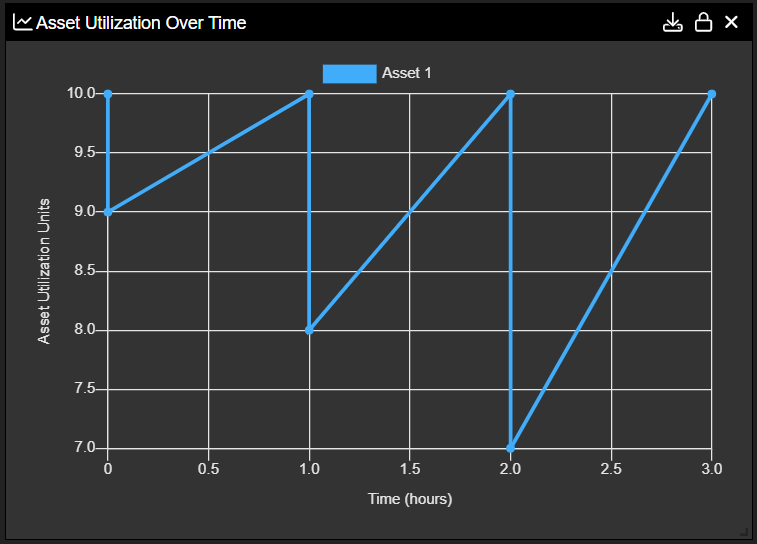Asset Utilization will capture an ‘Asset’ once an ‘Action’ has begun execution. The usage of each ‘Asset’ can be monitored during the simulation and graphically displayed in the simulation results as shown above. To control simulation flow, each ‘Asset’ utilized must have a parent ‘Asset.’  Typically, an Innoslate model will have a Physical Hierarchy of ‘Assets.’ ‘Assets’ in this hierarchy have a Multiplicity relationship attribute. If the ‘Asset’ is part of this hierarchy and the Multiplicity relationship attribute is set then the ‘Asset’ can be utilized.

In the image below, this is the Entity View for Asset 1 which is what will be tracked in the simulator. As an example, we will only track one Asset know as Asset 1. Asset 1's Entity View shows it's Parent entity, Parent Asset 1. As you can see below in the indicated red box, Parent Asset 1 has a multiplicity of 10. This fulfills the above steps italicized.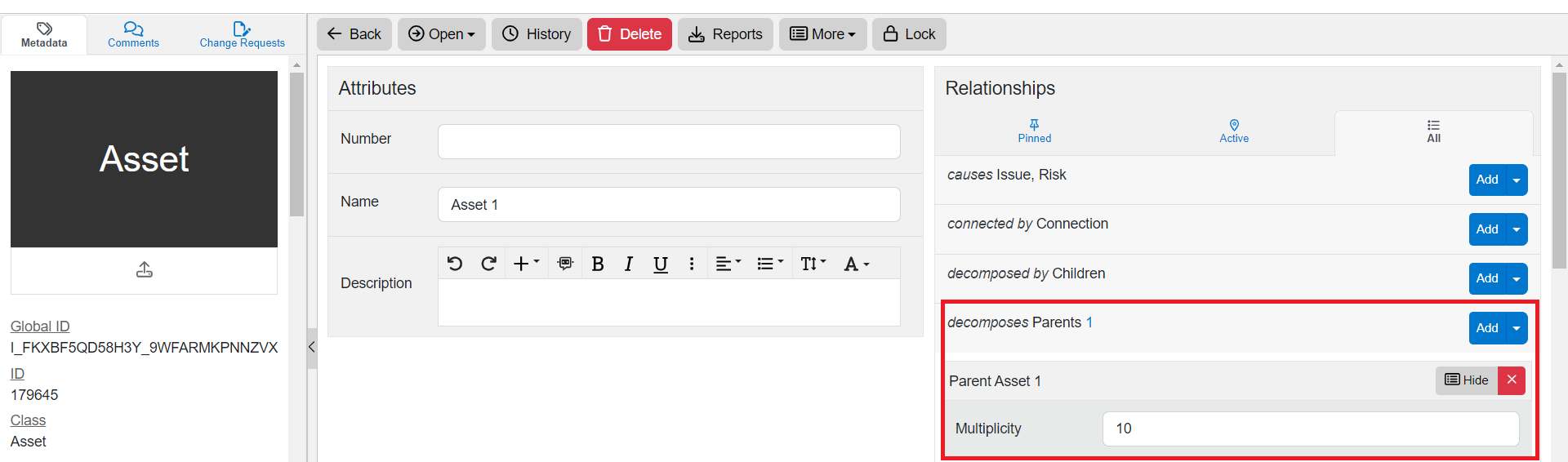The below Action Diagram is what will be simulated: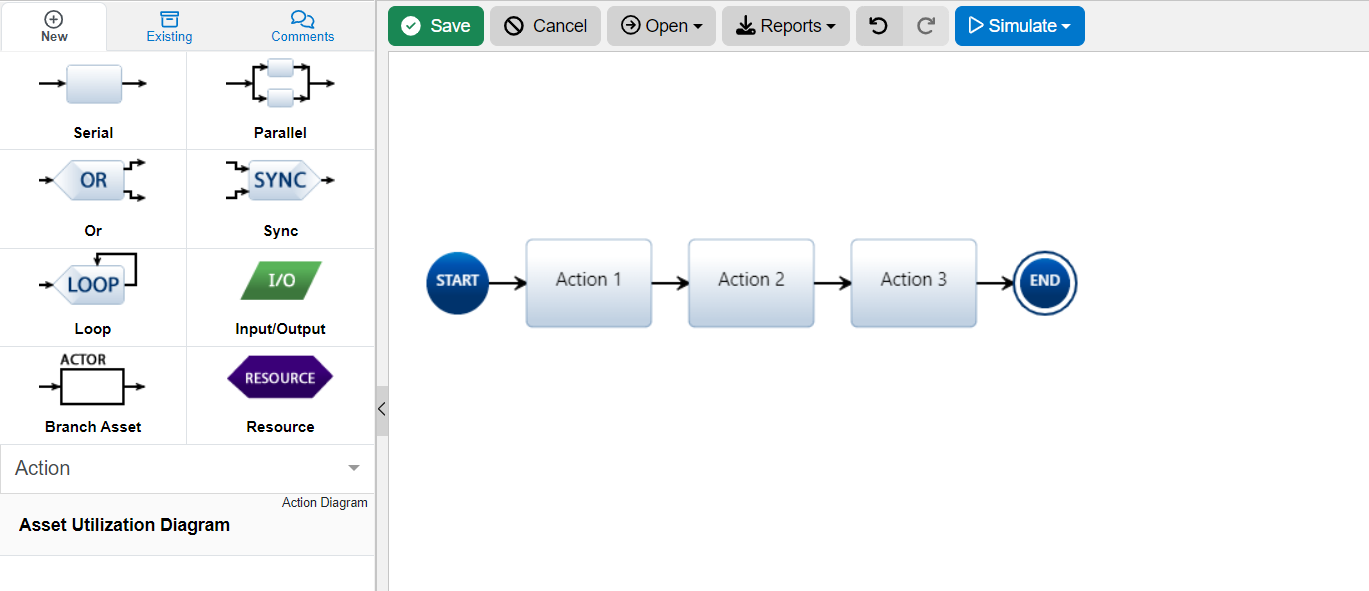To utilize an ‘Asset,’ set the Amount attribute on the performed by relationship.

As explained above, the amount of Assets indicated for each Action is indicated in the red box below. This indicates Action 1 takes 1 of Asset 1, Action 2 takes 2 of Asset 1, and Action 3 takes 3 of Asset 1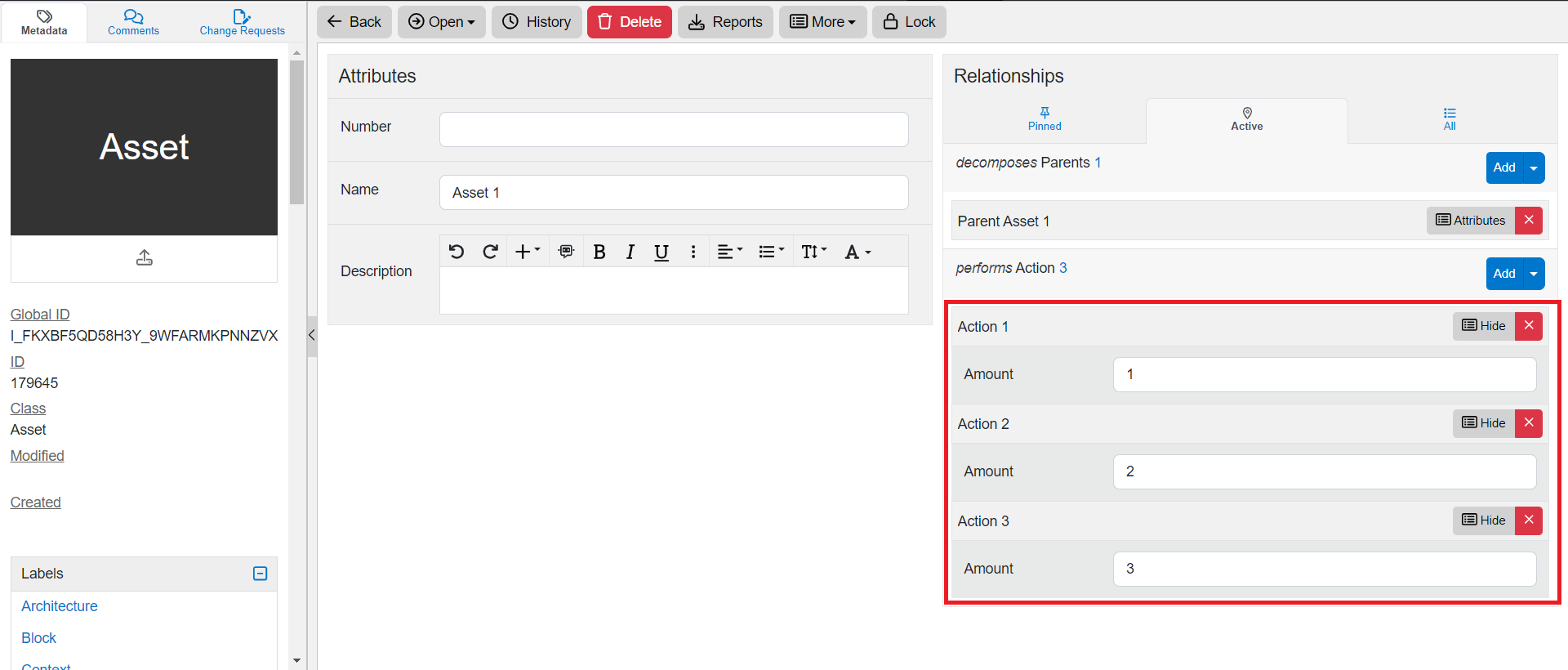Since the Parent Asset's relationship attribute 'Multiplicity'  is set to 10, this means that in the simulator, you'll see the Asset Utilization Over Time widget start at 10 and once each action starts, it will take the corresponding amount from the Parent Asset 1 Multiplicty total (10).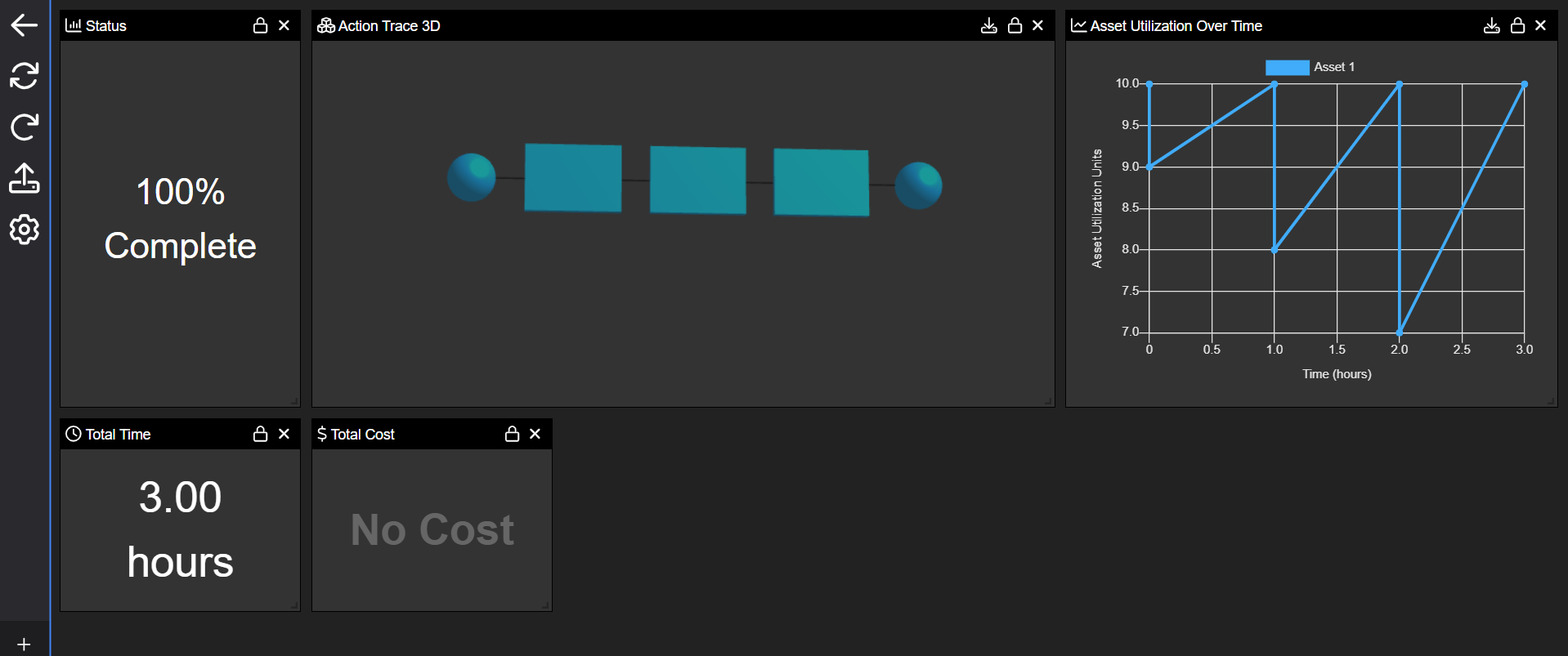Above is the end result of the Action Diagram's simulation. Note the total time was 3 hours, indicating each Action's Duration is set to 1 hour. That is important to note since it is Asset Utilization Over Time.

Below is the Asset Utilization Over Time widget magnified. The asset units are on the Y-axis and the time is on the X-axis. You can see the Parent Asset's Multiplicity starting at 10 in the widget and as Action 1 started, it took 1 asset away from the 10 so it moved to 9. Then as the simulator moved on to Action 2, it took 2 from the 10 so it moved to 8. Lastly, Action 3 took 3 from the 10 so it went down to 7. Since there are no more actions in the diagram to simulate, it ends back on 10.Note, the graph will reflect the proper numbering on the X & Y-axis as applied to your model.